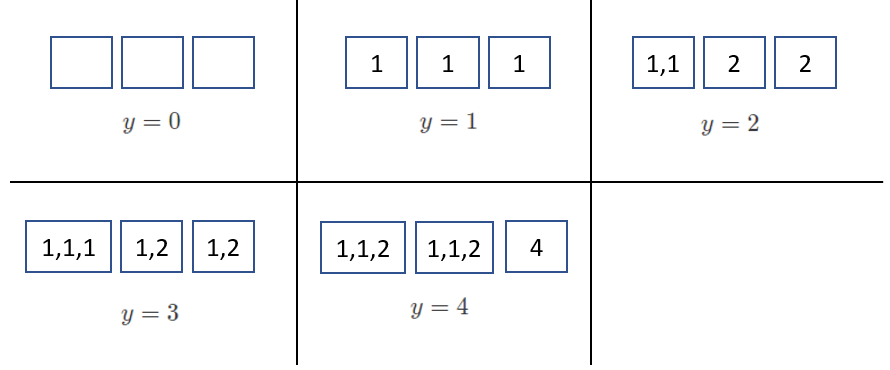시간 제한 메모리 제한 제출 정답 맞은 사람 정답 비율
1 초 1024 MB 86 26 24 30.380%

## 문제

Aunty Khong is organising a competition with $x$ participants, and wants to give each participant a bag of biscuits. There are $k$ different types of biscuits, numbered from $0$ to $k-1$. Each biscuit of type $i$ ($0 \leq i \leq k-1$) has a tastiness value of $2^i$. Aunty Khong has $a[i]$ (possibly zero) biscuits of type $i$ in her pantry.

Each of Aunty Khong's bags will contain zero or more biscuits of each type. The total number of biscuits of type $i$ in all the bags must not exceed $a[i]$. The sum of tastiness values of all biscuits in a bag is called the total tastiness of the bag.

Help Aunty Khong find out how many different values of $y$ exist, such that it is possible to pack $x$ bags of biscuits, each having total tastiness equal to $y$.

## 구현

You should implement the following proceedure:

int64 count_tastiness(int64 x, int64[] a)

• $x$: the number of bags of biscuits to pack.
• $a$: an array of length $k$. For $0 \leq i \leq k-1$, $a[i]$ denotes the number of biscuits of type $i$ in the pantry.
• The procedure should return the number of different values of $y$, such that Aunty can pack $x$ bags of biscuits, each one having a total tastiness of $y$.
• The procedure is called a total of $q$ times (see Constraints and Subtasks sections for the allowed values of $q$). Each of these calls should be treated as a separate scenario.

## 제한

• $1 \leq k \leq 60$
• $1 \leq q \leq 1000$
• $1 \leq x \leq 10^{18}$
• $0 \leq a[i] \leq 10^{18}$ (for all $0 \leq i \leq k-1$)
• For each call to count_tastiness, the sum of tastiness values of all biscuits in the pantry does not exceed $10^{18}$.

## 예시 1

Consider the following call:

count_tastiness(3, [5, 2, 1])


This means that Aunty wants to pack $3$ bags, and there are $3$ types of biscuits in the shop:

• $5$ biscuits of type $0$, each having a tastiness value $1$,
• $2$ biscuits of type $1$, each having a tastiness value $2$,
• $1$ biscuit of type $2$, each having a tastiness value $4$.

The possible values of $y$ are $[0, 1, 2, 3, 4]$. For instance, in order to pack $3$ bags of total tastiness $3$, Aunty can pack:

• one bag containing three biscuits of type $0$, and
• two bags, each containing one biscuit of type $0$ and one biscuit of type $1$.

Since there are $5$ possible values of $y$, the procedure should return $5$.## 예시 2

Consider the following call:

count_tastiness(2, [2, 1, 2])


This means that Aunty wants to pack $2$ bags, and there are $3$ types of biscuits in the shop:

• $2$ biscuits of type $0$, each having a tastiness value $1$,
• $1$ biscuits of type $1$, each having a tastiness value $2$,
• $2$ biscuits of type $2$, each having a tastiness value $4$.

The possible values of $y$ are $[0,1,2,4,5,6]$. Since there are $6$ possible values of $y$, the procedure should return $6$.

## 서브태스크

번호 배점 제한
1 9

$q \leq 10$, and for each call to count_tastiness, the sum of tastiness values of all biscuits in the pantry does not exceed $100\;000$.

2 12

$x = 1$, $q \leq 10$

3 21

$x \leq 10\;000$, $q \leq 10$

4 35

The correct return value of each call to count_tastiness does not exceed $200\;000$.

5 23

## 출처• 문제를 만든 사람: Mikhail Tikhomirov

## 제출할 수 있는 언어

C++17, C++14, C++20, C++14 (Clang), C++17 (Clang), C++20 (Clang)

## 채점 및 기타 정보

• 예제는 채점하지 않는다.